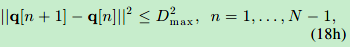# How can i write this constrain in cvx

x,y are two variables which are two vectors by N*1. [x y] is the coordinates of an moving object. Dmax is the longest distance that the object can travel within each time slot. So how can I write the following constrain?if not in CVX, I think the followling expression maybe right.

for i=1:N-1
(x(i+1)-x(i))^2+(y(i+1)-y(i))^2<=Dmax^2
end

But how can i write this in CVX?

`norm([x(i+1)-x(i),y(i+1)-y(i)]) <= Dmax`

I think you can eliminate the for loop and make this a little faster by using `norms` as in your previous question (x-x0).^2+(y-y0).^2 .

Problem solved, thanks! But I have another question. The output of square_pos(x) is 1/-1, for example: the output of square_pos(2) is 1, and the output of square_pos([1;2;3]) is [1;1;1]. I don’t know how the problem appears.

I checked the source code of square_pos(), and in fact square_pos() calls another function square.
`y = square( max( x, 0 ) );`
But the fuction square() doesn’t return the square of the input. It returns 1 or -1. So I guess this is where the problem arises. But how can I fix the problem?

Does the output of
`which -all square`
show anything other than two entries in cvx directories? If so, there is another `square` on your computer which perhaps is getting in ahead of CVX’s version. However, perhaps that is only a problem for numerical arguments and not for CVX arguments. The version inside the `@cvx` directory is what is used for CVX arguments, and that is more complicated than the version for numerical arguments.

The output of `which -all square` is:

which -all square
D:\matlab\toolbox\signal\signal\square.m
D:\matlab\bin\cvx\functions@cvx\square.m % cvx method

So there are two square functions, and `square_pos()` calls the first one. How can I let `square_pos()` call the CVX’s version of `square()` ?

I suggest you get the other `square` out of the MATLAB path, or at least after CVX’s version.

As to whether that will screw up the signal processing toolbox functionality …?

Thanks! I’ll change the MATLAB path to see if that works.

I changed my MATLAB path, and this time the CVX version `square` is called succesively. But here is another error: `The function 'square' corresponding to the input parameter of type 'double' is not defined`

The best information available on the subject is from the CVX developer, mcg, in the thread Warning about `square.m` during CVX installation .

I can;t offer any help on this beyond that.

Okay, Thank you Mark!